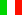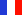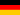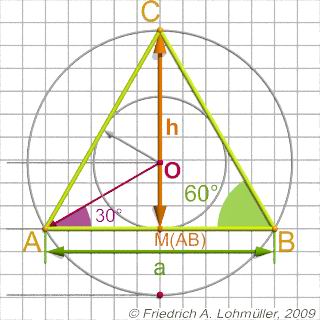###### Descriptions and Examples for the POV-Ray Raytracerby Friedrich A. Lohmüller     Elementary Geometry for Raytracing
ItalianoFrançaisDeutschHome
- POV-Ray Tutorial

- Geometrical Basics
for Raytracing

Right-angled Triangle
Pythagorean Theorem
Trigonometry Basics
Law of cosines
Equilateral Triangle
Regular Polygon
Polyhedron
Tetrahedron
Octahedron
Cube & Cuboid
Dodecahedron
Icosahedron
Cuboctahedron
Truncated Octahedron
Rhombicuboctahedron
Truncated Icosahedron
Circles
Tangent circles
Internal Tangents
External Tangents

- Geometric 3D Animations

## Equilateral TriangleIn the following we write for the square root of a number the expression "sqrt(ZAHL)" conforming to the syntax used in POV-Ray.Note: To avoid any collision with built-in identifiers and reserved words in POV-Ray, it's strongly recommanded to use only words beginning with capital letters for all identifiers of variables declared by the user, i.e. use "Ri" instead of "r" and use "H" instead of "h".

 Dimensions Length of triangle base side: a. All internal angles: 60° The height of an equilateral triangle: h = 1/2 * sqrt(3) * a ; or h = sin (radians(60)) * a ; The height of the incenter O or the incircle radius of an equilateral triangle: r = 1/6 * sqrt(3) * a ; The radius of circumcircle of an equilateral triangle: R = 1/3 * sqrt(3) * a ;An equilateral triangle
top

 © Friedrich A. Lohmüller, 2009 http://www.f-lohmueller.de